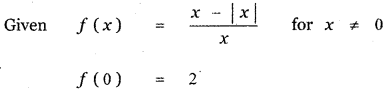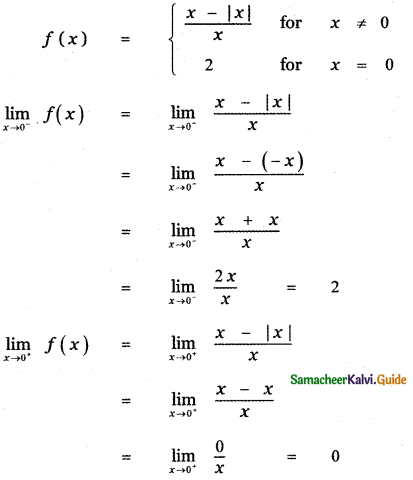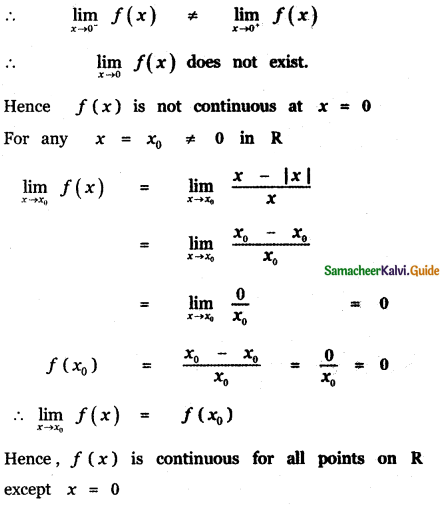Tamilnadu State Board New Syllabus Samacheer Kalvi 11th Maths Guide Pdf Chapter 9 Limits and Continuity Ex 9.6 Text Book Back Questions and Answers, Notes.

## Tamilnadu Samacheer Kalvi 11th Maths Solutions Chapter 9 Limits and Continuity Ex 9.6

Choose the correct or the most suitable answer

Question 1.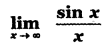(1) 1
(2) 0
(3) ∞
(4) -∞
(2) 0

Explaination: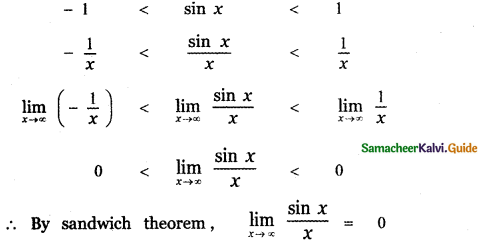Question 2.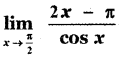(1) 2
(2) 1
(3) -2
(4) 0
(3) -2

Explaination: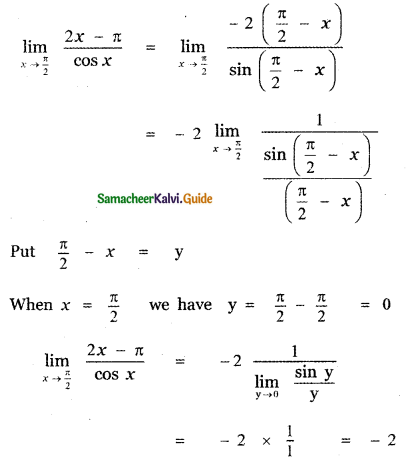Question 3.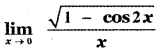(1) 0
(2) 1
(3) √2
(4) does not exist
(3) √2

Explaination: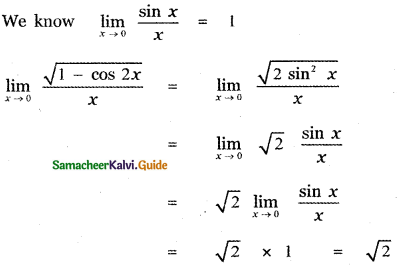Question 4.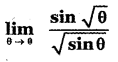(1) 1
(2) -1
(3) 0
(4) 2
(1) 1

Explaination: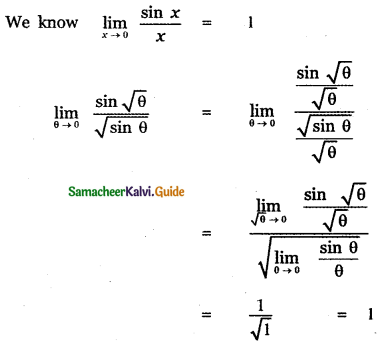Question 5.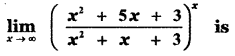(1) e4
(2) e2
(3) e3
(4) 1
(1) e4

Explaination: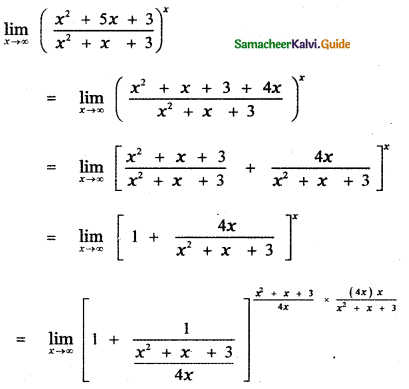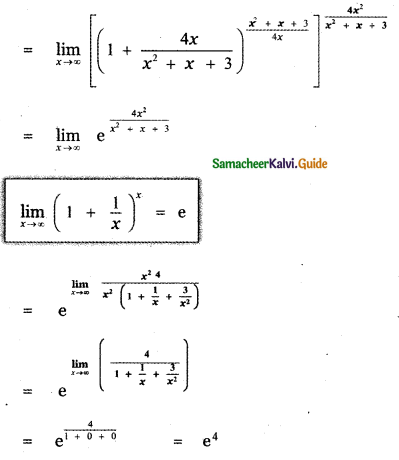Question 6.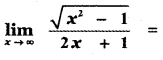(1) 1
(2) 0
(3) -1
(4) $$\frac{1}{2}$$
(4) $$\frac{1}{2}$$

Explaination: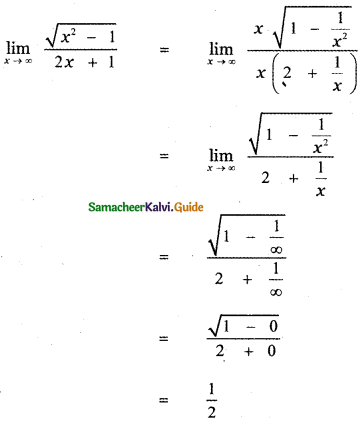Question 7.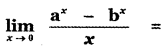(1) log ab
(2) log $$\left(\frac{\mathbf{a}}{\mathbf{b}}\right)$$
(3) log $$\left(\frac{\mathbf{b}}{\mathbf{a}}\right)$$
(4) $$\frac{\mathbf{a}}{\mathbf{b}}$$
(2) log $$\left(\frac{\mathbf{a}}{\mathbf{b}}\right)$$

Explaination: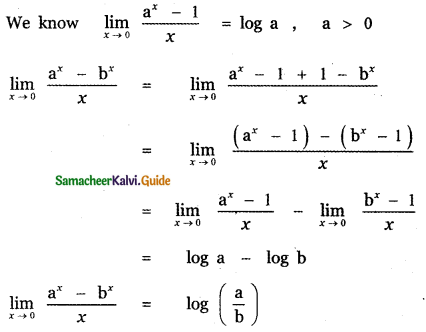Question 8.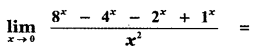(1) 2 log 2
(2) 2(log 2)2
(3) log 2
(4) 3 log 2
(2) 2(log 2)2

Explaination: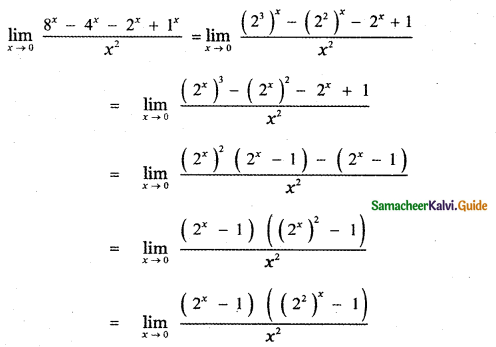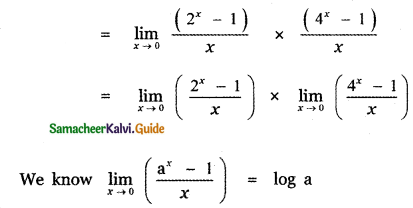= (log 2) × (log 4)
= log 2 × log 22
= log 2 × 2 log 2
= 2 (log 2)2Question 9.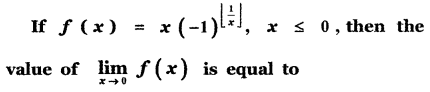(1) -1
(2) 0
(3) 2
(4) 4
(2) 0

Explaination: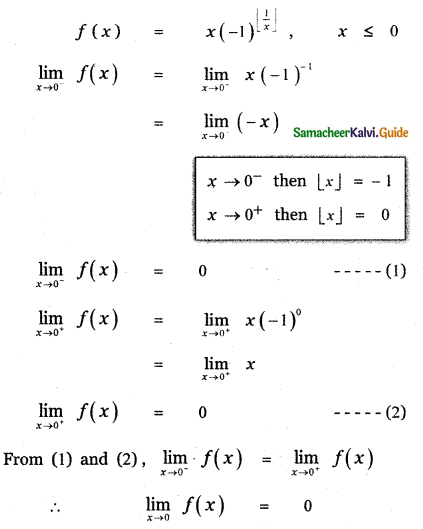Question 10.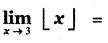(1) 2
(2) 3
(3) does not exist
(4) 0
(3) does not exist

Explaination: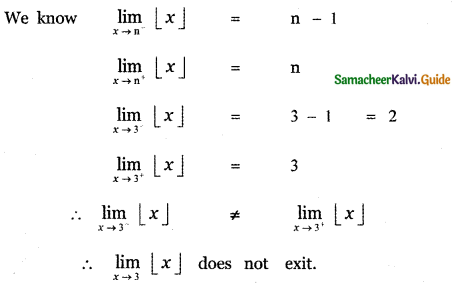Question 11.
Let the function f be defined by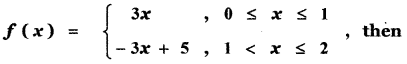(1)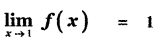(2)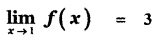(3)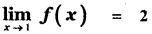(4)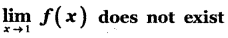(4)Explaination: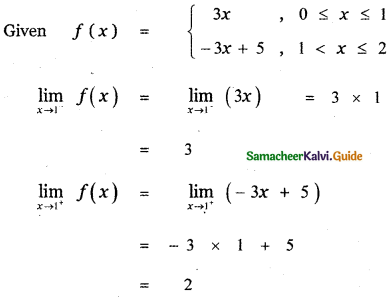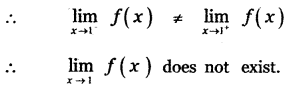Question 12.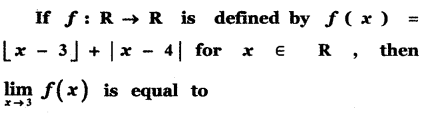(1) -2
(2) -1
(3) 0
(4) 1
(3) 0

Explaination: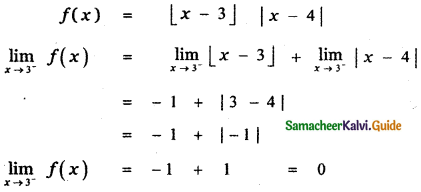Question 13.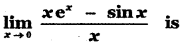(1) 1
(2) 2
(3) 3
(4) 0
(4) 0

Explaination: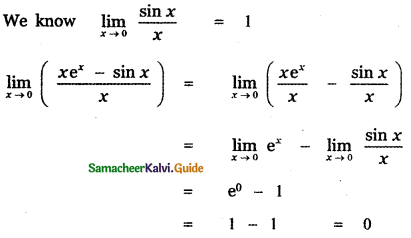Question 14.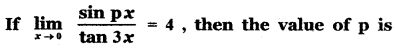(1) 6
(2) 9
(3) 12
(4) 4
(3) 12

Explaination: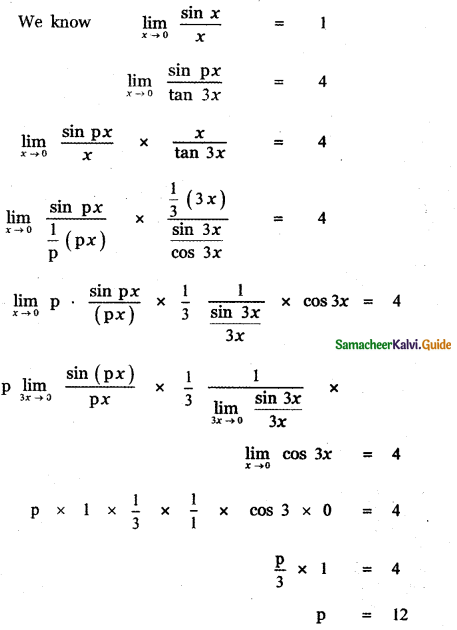Question 15.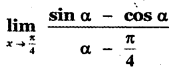(1) $$\sqrt{2}$$
(2) $$\frac{1}{\sqrt{2}}$$
(3) 1
(4) 2
(1) $$\sqrt{2}$$

Explaination: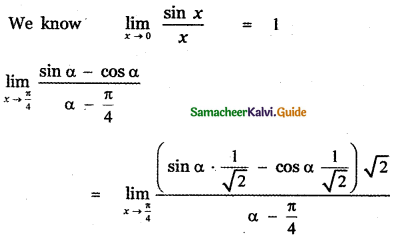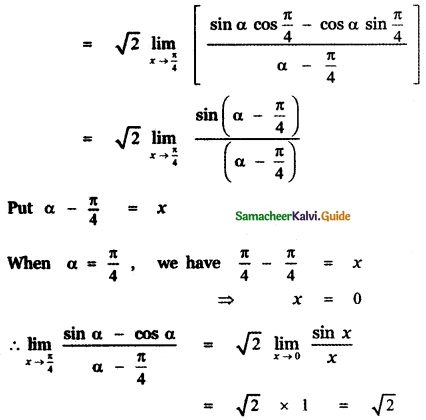Question 16.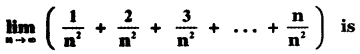(1) $$\frac{1}{2}$$
(2) 0
(3) 1
(4) ∞
(1) $$\frac{1}{2}$$

Explaination: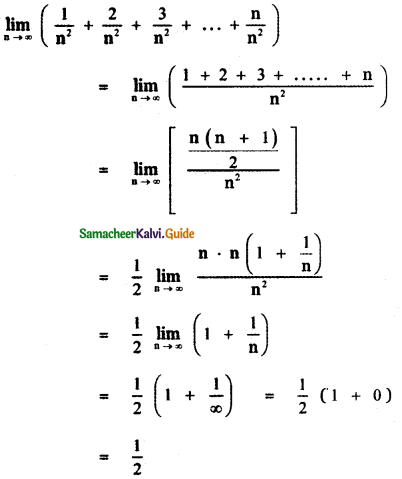Question 17.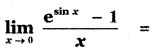(1) 1
(2) e
(3) $$\frac{1}{\mathrm{e}}$$
(4) 0
(1) 1

Explaination: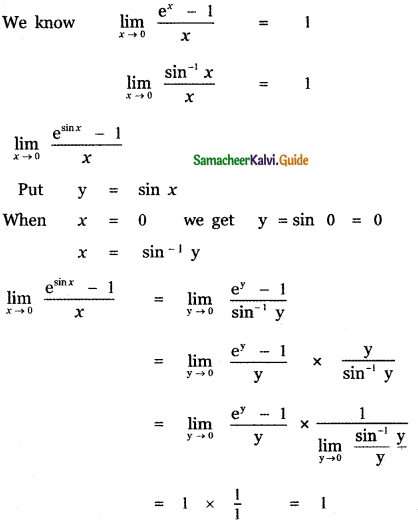Question 18.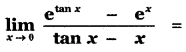(1) 1
(2) e
(3) $$\frac{1}{2}$$
(4) 0
(1) 1

Explaination: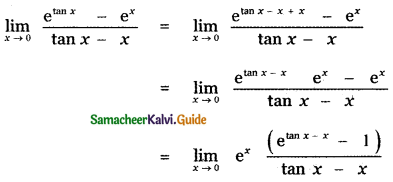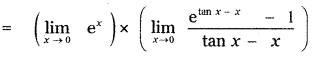Put tan x – x = y
When x = 0, tan 0 – 0 = y
0 = y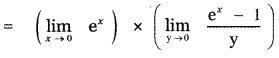= e0 × 1 = 1 × 1 = 1Question 19.
The value of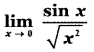is
(1) 1
(2) -1
(3) 0
(4) ∞
(1) 1

Explaination: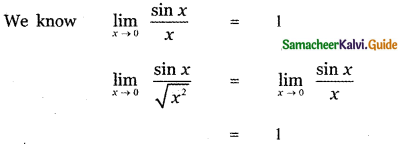Question 20.
The value of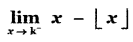, where k is an integer is
(1) -1
(2) 1
(3) 0
(4) 2
(2) 1

Explaination: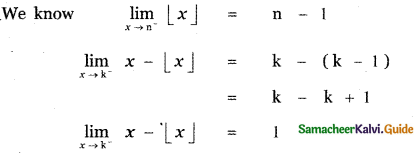Question 21.
At x = $$\frac{3}{2}$$ the function f(x) = $$\frac{|2 x-3|}{2 x-3}$$ is
(1) continuous
(2) discontinuous
(3) differentiable
(4) non zero
(2) discontinuous

Explaination:
f(x) = $$\frac{|2 x-3|}{2 x-3}$$
f(x) is not defined at x = $$\frac{3}{2}$$
∴ f(x) is discontinuous at x = $$\frac{3}{2}$$

Question 22.
Let f : R → R be defined by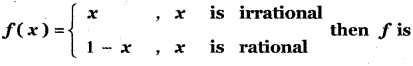(1) discontinuous x = $$\frac{1}{2}$$
(2) continuous x = $$\frac{1}{2}$$
(3) continuous everywhere
(4) discontinuous everywhere
(2) continuous x = $$\frac{1}{2}$$

Explaination: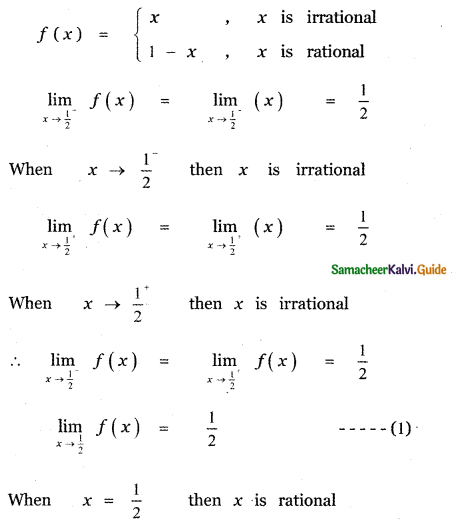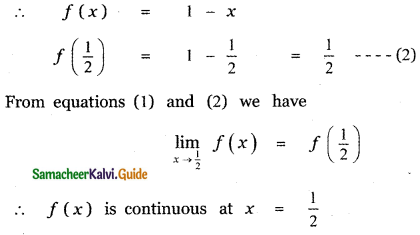Question 23.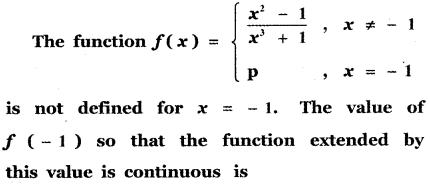(1) $$\frac{2}{3}$$
(2) –$$\frac{2}{3}$$
(3) 1
(4) 0
(2) –$$\frac{2}{3}$$

Explaination: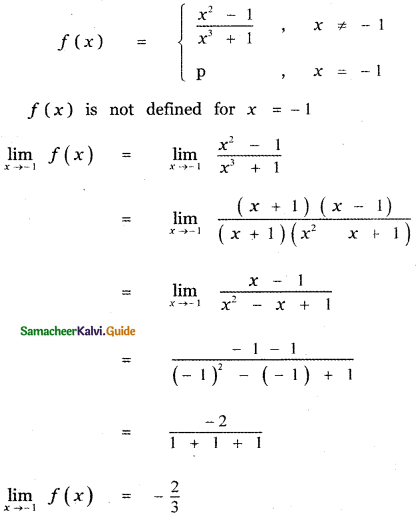At = -1, f(x) has a removable discontinuity Redefining f(x) as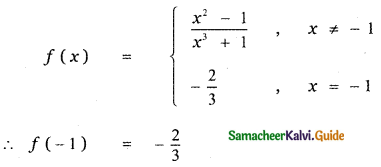Question 24.
Let f be a continuous function on [2, 5]. If f takes only rational values for all x and f(3) = 12, then f (4.5) is equal to
(1)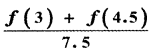(2) 12
(3) 17.5
(4)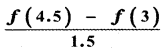(2) 12

Explaination:
Given f(3) = 12
f takes only rational values
f(x) = 12
f(3) = 12
f(4.5) = 12Question 25.
Let a function f be defined by f(x) =  for x ≠ 0 and f(0) = 2. Then f is
(1) continuous nowhere
(2) continuous everywhere
(3) continuous for all x except x = 1
(4) continuous for all x except x = 0